Next: Block-Oriented Iteration Up: Join Strategies Previous: Join Strategies

Simple Iteration

1. If we don't create an index, we must examine every pair of tuples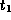in deposit and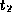in customer. This means examining 10,000 * 200 = 2,000,000 pairs!
2. If we execute this query cleverly, we can cut down the number of block accesses. We use the following method:

for each tuple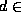deposit do

begin

for each tuple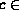customer do

begin

examine pair (d, c) to see if a

tuple should be added to the result

end

end

• We read each tuple of deposit once.
• This could require 10,000 block accesses.
• The total number of block access, if the tuples are not stored together physically, would be 10,000 + 10,000 * 200 = 2,010,000.
• If we put customer in the outer loop, we get 2,000,200 accesses.
• If the tuples of deposit are stored together physically, fewer accesses are required (at 20 per block, 10,000/20 = 500 block accesses).
• We read each tuple of customer once for each tuple of deposit.
• This suggests we read each tuple of customer 10,000 times, giving as many as 2,000,000 accesses to read customer tuples!
• This would give a total of 2,000,500 accesses.
• We can reduce accesses significantly if we store customer tuples together physically.
• At 20 tuples per block, only 10 accesses are required to read the entire relation (as opposed to 200).
• Then we only need 10 * 10,000 = 100,000 block accesses for customer.
• This gives a total of 100,500.
3. Text says further savings are possible if we use customer in the outer loop.
• Now we reference each tuple of deposit once for each tuple of customer.
• If deposit tuples are stored together physically, then since 20 tuples fit on one block,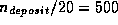accesses are needed to read the entire relation.
• Since customer has 200 tuples, we read the deposit relation 200 times.
• Earlier printings of the text say at most 200 * 500 = 10,000 block accesses to deposit are required.
• WRONG! 200 * 500 is 100,000.
• Total cost is then 100,000 for inner loop plus 10 accesses to read the customer relation once for a total of 100,010.
• Compared to previous estimate of 100,500, the savings are small (490).
4. Note that we are considering worst-case number of block reads, where every time a block is needed it is not in the buffer.

Good buffer management can reduce this considerably.Next: Block-Oriented Iteration Up: Join Strategies Previous: Join Strategies

Osmar Zaiane
Sun Jul 26 17:45:14 PDT 1998